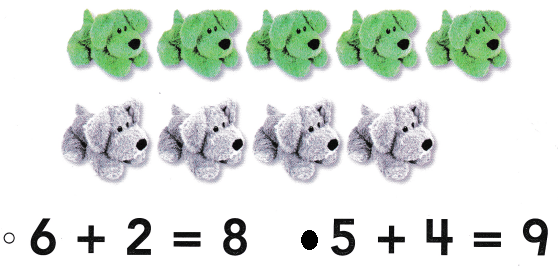# Texas Go Math Kindergarten Lesson 13.3 Answer Key Sums Up to 9

Refer to our Texas Go Math Kindergarten Answer Key Pdf to score good marks in the exams. Test yourself by practicing the problems from Texas Go Math Kindergarten Lesson 13.3 Answer Key Sums Up to 9.

## Texas Go Math Kindergarten Lesson 13.3 Answer Key Sums Up to 9

Essential Question
How you show and write addition sentences for sums to 9?

Explore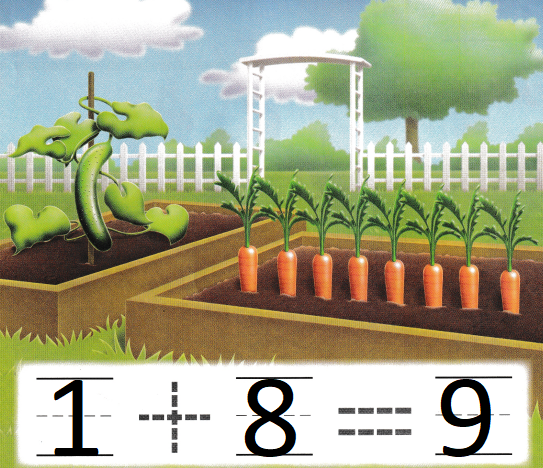Explanation:
1 cucumber and 8 carrots
1 + 8 = 9
The sum of 1 and 8 is 9

Directions
1-3. Listen to the addition word problem. Complete the addition sentence. Trace the symbols.

Share and Show

Question 1.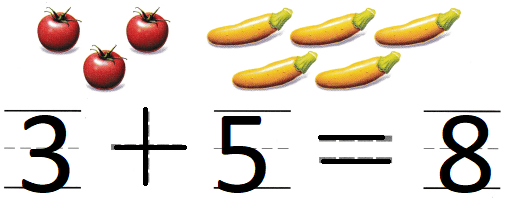Explanation:
3 + 5 = 8
The sum of 3 and 5 is 8

Question 2.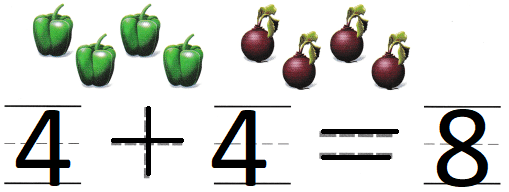Explanation:
4 capsicum and 4 turnips
4 + 4 = 8
The sum of 4 and 4 is 8

Question 3.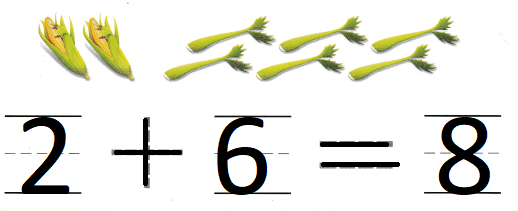Explanation:
2 corns and 6 lattice
2 + 6 = 8
The sum of 2 and 6 is 8

Directions

Question 4.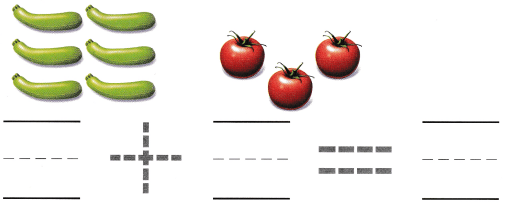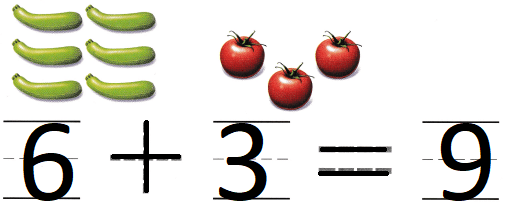Explanation:
6 bottle guards and 3 tomatoes
6 + 3 = 9
the sum of 6 and 3 is 9

Question 5.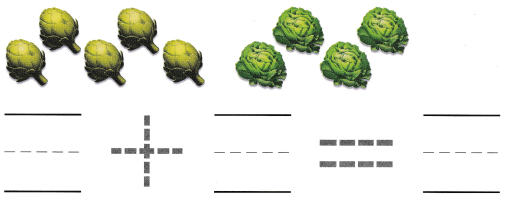Explanation:
5 Artichoke and 4 cabbage
5 + 4 = 9
The sum of 5a nd 4 is 9

Question 6.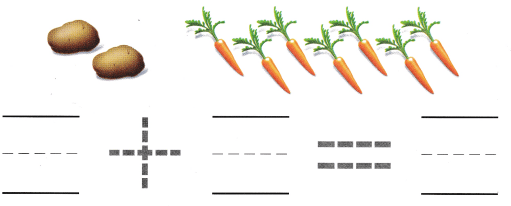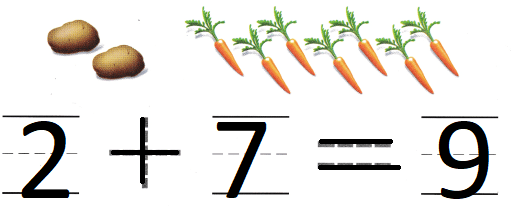Explanation:
2 potatoes and 7 carrots
2 + 7 = 9
The sum of 2 and 7 is 9

Directions

Home Activity

• Have your child use his or her fingers on two bonds to show two numbers and tell how many in all.

Problem Solving

Question 7.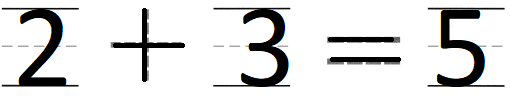Explanation:
In one hand there are 2 fingers open
and in the other hand there are 3 fingers open
2 + 3 = 5
so, The sum of 2 and 3 is 5

Question 8.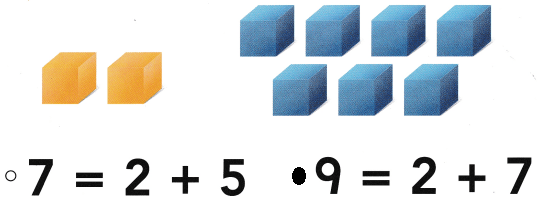Explanation:
2 yellow boxes and 7 blue boxes
2 + 7 = 9
The sum of 2 and 7 is 9

Directions
7. Shelby has nine counters. None of them are blue. How many are red? Draw the counters. Complete the addition sentence. 8. Choose the correct answer. What does the picture show?

### Texas Go Math Kindergarten Lesson 13.3 Homework and Practice

Question 1.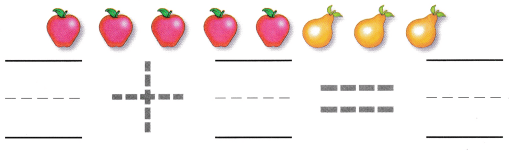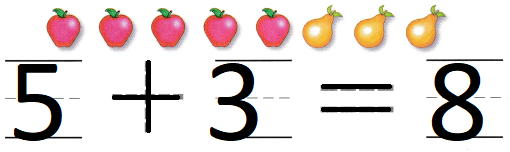Explanation:
Mr. Gates bought 5 apples and 3 pears.
5 + 3 = 8
8 pieces of fruit that he buy

Question 2.Explanation:
Mr. Jones eats 2 strawberries and 6 blueberries.
2 + 6 = 8
8 berries that he eat

Question 3.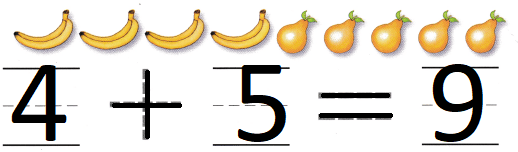Explanation:
There are 4 bananas and 5 pears in a bowl.
4 + 5 = 9
9 pieces of fruit are there

Directions
Complete the addition sentence. 1. Mr. Gates bought 5 apples and 3 pears. How many pieces of fruit did he buy? 2. Mr. Jones eats 2 strawberries and 6 blueberries. How many berries does he eat? 3. There are 4 bananas and 5 pears in a bowl. How many pieces of fruit are there?

Texas Test Prep

Lesson Check

Question 4.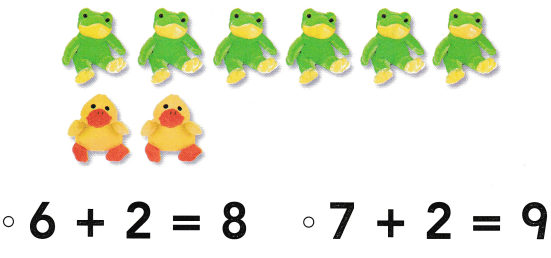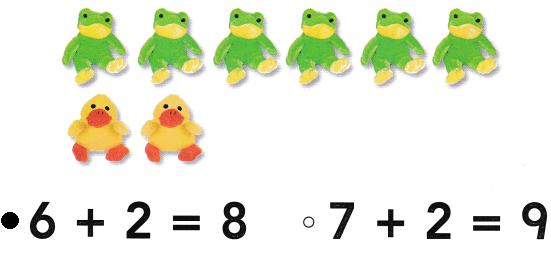Explanation:
6 frog toys and 2 duck toys
6 + 2 = 8
There are 8 toys in all.

Question 5.